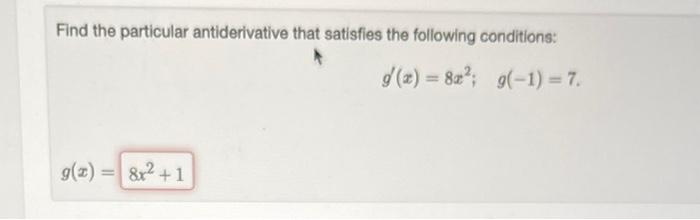Home / Expert Answers / Calculus / find-the-particular-antiderivative-that-satisfies-the-following-conditions-g-prime-x-8-x-2-pa947

# (Solved): Find the particular antiderivative that satisfies the following conditions: $g^{\prime}(x)=8 x^{2 ...Find the particular antiderivative that satisfies the following conditions: \[ g^{\prime}(x)=8 x^{2} ; \quad g(-1)=7$

We have an Answer from Expert